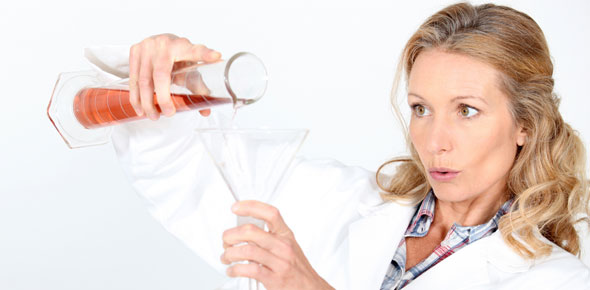# Chemical Reaction Of Respiration Quiz

21 Questions | Attempts: 248SettingsIn this quiz, you will get to learn about the chemical reactions. Play this quiz and test your knowledge on this!

• 1.
What type of reaction is FeS + 2HCl --> FeCl2 + H2S
• A.

Synthesis

• B.

Decomposition

• C.

Physical

• D.

Replacement

• 2.
In a chemical equation numbers often appear in front of a chemical formula.  These numbers tell you the
• A.

Number of atoms in each molecule in the reaction

• B.

Number of elements in the reaction

• C.

Number of molecules or atoms of each substance in the reaction

• D.

Number of molecules in each atom in the reaction

• 3.
Chemistry is...
• A.

A characteristic of substance that can be observed without changing the substance into another substance

• B.

The study of matter an how matter changes

• C.

Anything that has mass and takes up space

• D.

A rapid reaction between oxygen and a substance called a fuel

• 4.
A chemical reaction that absorbs energy in the form of heat is described as
• A.

Endothermic

• B.

Exothermic

• C.

Combustion

• D.

Unbalanced

• 5.
The substances listed on the left side of a chemical equation are the
• A.

Products

• B.

Coefficients

• C.

Precipitates

• D.

Reactants

• 6.
Which two processes could be considered opposites?
• A.

Decomposition and replacement

• B.

Replacement and precipitate

• C.

Precipitate and synthesis

• D.

Synthesis and decomposition

• 7.
Which group contains the most elements?
• A.

Metalloids

• B.

Nonmetals

• C.

Metals

• D.

Transition elements

• 8.
Every chemical reaction involves a change in
• A.

Mass

• B.

Energy

• C.

Concentration

• D.

State

• 9.
The number placed below an element's symbol in a chemical formula is called a
• A.

Subscript

• B.

Coefficient

• C.

Reactant

• D.

Product

• 10.
CaCO3  represents a chemical ...
• A.

Symbol

• B.

Formula

• C.

Subscript

• D.

Reaction

• 11.
The only sure evidence for a chemical reaction is
• A.

The formation of a gas

• B.

A color change

• C.

The production of one or more new substances

• D.

Changes in properties

• 12.
The principle of conservation of mass is true...
• A.

Only for reactions that take place in open systems

• B.

Only for reactions that take place in closed systems

• C.

For all reactions

• D.

For no reactions

• 13.
What happens when chemical bonds break and new bonds form
• A.

A physical change

• B.

A chemical reaction

• C.

Matter is destroyed

• D.

Surface area increases

• 14.
A chemical equation that shows the same number of each kind of atom on both sides of the equation is said to be....
• A.

Balanced

• B.

Unbalanced

• C.

An incomplete reaction

• D.

A replacement reaction

• 15.
From an element's location in the periodic table you can predict
• A.

Its properties

• B.

Name

• 16.
In chemical reactions, what does the principle of conservation of mass mean?
• A.

Matter is not created or destroyed

• B.

The total mass of the reactants is greater than the total mass of the products

• C.

The total mass of the reactants is less than the total mass of the products

• D.

Matter can never be changed

• 17.
What type of chemical reaction is represented in the reaction            ____ N2 + __3__ H2 à ___2_ NH3
• A.

Synthesis

• B.

Decomposition

• C.

Replacement

• D.

Combustion

• 18.
2 H2 +  O2 à ____ H2O
• 19.
2  Ag2O à ____ Ag +  O2
• 20.
What are the reactants in the following equations           2   H2O + O2 à 2 H2O2
• A.

Water and oxygen

• B.

Hydrogen peroxide

• C.

Carbon dioxide

• D.

Oxygen

## Related TopicsBack to top
×

Wait!
Here's an interesting quiz for you.